# Multiply Matrix by Vector in R

• Last Updated : 26 Mar, 2021

A matrix is a 2-dimensional structure whereas a vector is a one-dimensional structure. In this article, we are going to multiply the given matrix by the given vector using R Programming Language. Multiplication between the two occurs when vector elements are multiplied with matrix elements column-wise.

Approach:

• Create a matrix
• Create a vector
• Multiply them
• Display result.

Method 1: Naive method

Once the structures are ready we directly multiply them using the multiplication operator(*).

Example:

## R

 `# create a vector for matrix elements``vector1=``c``(1,2,3,4,5,6,7,8,9,10,11,12)`` ` `# Create A matrix with 2 rows and 6 columns``matrix1 <- ``matrix``(vector1, nrow=2,ncol=6) `` ` `# multiplication vector``mul_vec=``c``(1,2,3,4)`` ` `# print multiplication result``print``(matrix1*mul_vec)`

Output: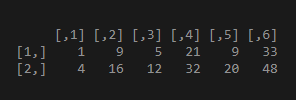Example 2:

## R

 `# create a vector for matrix elements``vector1=``c``(1,2,3,4,5,6,7,8,9,10,11,12,13,14,15,16)`` ` `# Create A matrix with 4 rows and 4 columns``matrix1 <- ``matrix``(vector1, nrow=4,ncol=4) ``print``(matrix1)`` ` `mul_vec=``c``(1,2,3,4)`` ` `print``(``"Result"``)``print``(matrix1*mul_vec)`

Output: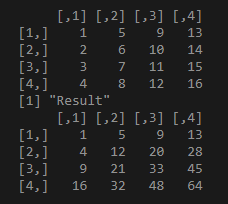Example 3:

This code has both matrix and vector has equal size

## R

 `# create a vector for matrix elements``vector1=``c``(1,2,3,4)`` ` `# Create A matrix with 2 rows and 2 columns``matrix1 <- ``matrix``(vector1,nrow=2,ncol=2) ``print``(matrix1)`` ` `mul_vec=``c``(1,2,3,4)`` ` `print``(``"Result"``)``print``(matrix1*mul_vec)`

Output: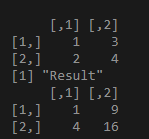Method 2: Using sweep()

we can use sweep() method to multiply vectors to a matrix. sweep() function is used to apply the operation “+ or – or ‘*’ or ‘/’ ” to the row or column in the given matrix.

Syntax:

sweep(data, MARGIN,  FUN)

Parameter:

• data=input matrix
• MARGIN: MARGIN = 2 means row; MARGIN = 1 means column.
• FUN: The operation that has to be done (e.g. + or – or * or /)

Here, we are performing “*” operation

Example:

## R

 `# create matrix with 15 elements``matrix1 <- ``matrix``(``c``(1,2,3,4,5,6,7,8,9,10,11,12,13,14,15),``                  ``nrow=3,byrow=``TRUE``)``print``(matrix1)``print``(``"---------------"``)`` ` `# create a vector``vector1 <- ``c``(1,2,3,4,5)`` ` `# apply sweep operation that multiplies row ``# wise(margin=2)``print``(``sweep``(matrix1, MARGIN=2,vector1, `*`))``print``(``"---------------"``)`` ` `# create elements with vector 2``vector2 <- ``c``(1,2,3)`` ` `# apply sweep operation that multiplies column``# wise with vector 2(margin=1)``print``(``sweep``(matrix1, MARGIN=1,vector2, `*`))`

Output: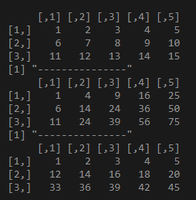Example 2:

## R

 `# create matrix with 8 elements``matrix1 <- ``matrix``(``c``(1,2,3,4,5,6,7,8),``                  ``nrow=2,byrow=``TRUE``)``print``(matrix1)``print``(``"---------------"``)`` ` `# create a vector``vector1 <- ``c``(1,2,3,4)`` ` `# apply sweep operation that multiplies ``# row wise(margin=2)``print``(``sweep``(matrix1, MARGIN=2,vector1, `*`))``print``(``"---------------"``)`` ` `# create elements with vector 2``vector2 <- ``c``(1,2)`` ` `# apply sweep operation that multiplies column ``# wise with vector 2(margin=1)``print``(``sweep``(matrix1, MARGIN=1,vector2, `*`))`

Output: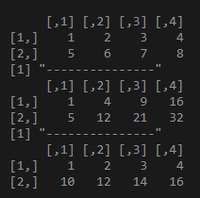My Personal Notes arrow_drop_up